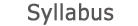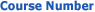0365-1800 Introduction to Computers for Statisticians2019 - 2020View groups
Course description

This is a survey course introducing basic consepts of programming using the R lanugague.

The following topics will be discussed:

• Introduction to R and RStudio
• Variables types
• Operations on variables
• Types of missing values (NA, NULL, NaN).
• Intro to functions and algorithms
• Flow control (if-else, loops, and vectoric operations)
• Recursion
• Run time complexity (big O notation)
• Algorithms for sorting and searching
• Lists, attributes, names
• Complex data structures (factor, matrix, data.frame)
• Aggregation methods (sapply, lapply, tapply, apply)